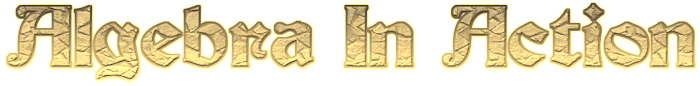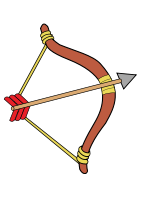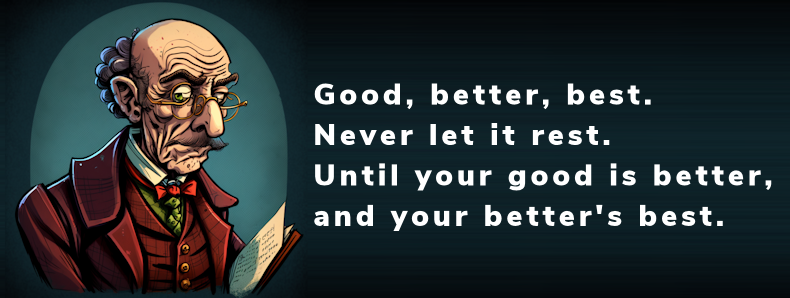### Real life problems adapted from an old Mathematics textbook (A First Book in Algebra, by Wallace C. Boyden 1895) which can be solved using algebra and common sense!

##### MenuLevel 1Level 2Level 3Level 4Level 5Level 6Level 7HelpMore

This is level 1: find two values given their ratio and either their sum or difference. You can earn a trophy if you get at least 9 questions correct and you do this activity online.

 1. The larger of two numbers is twice the smaller, and the sum of the numbers is 177. What is the smaller number?177 Working:2. A man bought a bow and arrow for £600, paying three times as much for the bow as for the arrow. How much did the bow cost?Working: £3. Two brothers, counting their money, found that together they had $378, and that John had five times as much as Charles. How much did John have? Working:$4. Divide the number 64 into two parts so that one part shall be seven times the other. What is the smaller part? Working:5. A man walked 24 miles in a day. If he walked twice as far in the morning as in the afternoon, how far did he walk in the afternoon? Working: miles6. For 72 cents Matthew bought some needles and thread, paying eight times as much for the thread as for the needles. How much did he pay for the thread? Working: cents7. In a school there are 672 pupils. If there are twice as many boys as girls, how many boys are there? Working:8. Find the first of two numbers such that their difference is 250 and the first is eleven times the other. Working:9. James gathered 12kg of nuts more than Henry gathered. What weight of nuts did Henry gather if James gathered three times as many as Henry? Working: kg10. A house cost £80000 more than a plot of land, and five times the cost of the plot equals the cost of the house. What was the cost of the house? Working: £11. Mr Meaner is 48 years older than his son, but he is only three times as old. How old is Mr Meaner? Working:12. The sum of two numbers is 4256, and one is 37 times the other. What is the larger number? Working:Check

This is Algebra In Action level 1. You can also try:
Level 2 Level 3 Level 4 Level 5 Level 6 Level 7## Instructions

Try your best to answer the questions above. Type your answers into the boxes provided leaving no spaces. As you work through the exercise regularly click the "check" button. If you have any wrong answers, do your best to do corrections but if there is anything you don't understand, please ask your teacher for help.

When you have got all of the questions correct you may want to print out this page and paste it into your exercise book. If you keep your work in an ePortfolio you could take a screen shot of your answers and paste that into your Maths file.

## Transum.org

This web site contains over a thousand free mathematical activities for teachers and pupils. Click here to go to the main page which links to all of the resources available.## More Activities:

Mathematicians are not the people who find Maths easy; they are the people who enjoy how mystifying, puzzling and hard it is. Are you a mathematician?

Comment recorded on the 28 September 'Starter of the Day' page by Malcolm P, Dorset:

"A set of real life savers!!
Keep it up and thank you!"

Comment recorded on the 3 October 'Starter of the Day' page by Mrs Johnstone, 7Je:

"I think this is a brilliant website as all the students enjoy doing the puzzles and it is a brilliant way to start a lesson."

Each month a newsletter is published containing details of the new additions to the Transum website and a new puzzle of the month.

The newsletter is then duplicated as a podcast which is available on the major delivery networks. You can listen to the podcast while you are commuting, exercising or relaxing.

Transum breaking news is available on Twitter @Transum and if that's not enough there is also a Transum Facebook page.

##### Featured ActivityThe Transum Newsletter for October 2023 has just been published. Click on the image above to read about the latest developments on this site and try to solve the puzzle of the month. You can read the newsletter online or listen to it by downloading the podcast.

There are answers to this exercise but they are available in this space to teachers, tutors and parents who have logged in to their Transum subscription on this computer.

A Transum subscription unlocks the answers to the online exercises, quizzes and puzzles. It also provides the teacher with access to quality external links on each of the Transum Topic pages and the facility to add to the collection themselves.

Subscribers can manage class lists, lesson plans and assessment data in the Class Admin application and have access to reports of the Transum Trophies earned by class members.

Subscribe

## Go Maths

Learning and understanding Mathematics, at every level, requires learner engagement. Mathematics is not a spectator sport. Sometimes traditional teaching fails to actively involve students. One way to address the problem is through the use of interactive activities and this web site provides many of those. The Go Maths page is an alphabetical list of free activities designed for students in Secondary/High school.

## Maths Map

Are you looking for something specific? An exercise to supplement the topic you are studying at school at the moment perhaps. Navigate using our Maths Map to find exercises, puzzles and Maths lesson starters grouped by topic.

## Teachers

If you found this activity useful don't forget to record it in your scheme of work or learning management system. The short URL, ready to be copied and pasted, is as follows:

Alternatively, if you use Google Classroom, all you have to do is click on the green icon below in order to add this activity to one of your classes.

It may be worth remembering that if Transum.org should go offline for whatever reason, there is a mirror site at Transum.info that contains most of the resources that are available here on Transum.org.

When planning to use technology in your lesson always have a plan B!

Transum,

Sunday, January 26, 2014

"These questions have been adapted from 'A First Book in Algebra' by Wallace Boyden. They all are designed to encourage an algebraic solution by setting up an equation (or alternatively simultaneous equations) and solving it. Some of the questions could be classified under the topic of ratio.
In his introduction Wallace Boyden states 'Algebra is so much like arithmetic that all that you know about addition, subtraction, multiplication, and division, the signs that you have been using and the ways of working out problems, will be very useful to you in this study. There are two things the introduction of which really makes all the difference between arithmetic and algebra. One of these is the use of letters to represent numbers, and you will see in the following exercises that this change makes the solution of problems much easier.'."

I'm Not A Humanist But..., Planet Earth

Saturday, May 24, 2014

"On level 4 of the 'algebra in action' section, question 11 says:
"Divide the number 137 into three parts, such that the second is 3 more than the first, and the third five times the second. What is the third part?"
The answer is 100, but it was marked as being wrong, so I tried again, but with 20 (the second number) and 17 (the first number) and it marked 17 as being correct.

[Transum: Thank you so much for taking the time to highlight this error. You were indeed right and the error has now been corrected. Thank you so much.]"

Nick Staples, Waipahu Intermediate

Wednesday, August 18, 2021

"Is there a way these algebra in action activities can be made into printable worksheets please.

[Transum: Thanks for your feedback Nick. Most of the Transum online exercises can be printed directly from your browser. The input boxes transform into dotted lines for pupils to write their answers on and if the page is being printed by a Transum subscriber, the answers appear at the bottom of the worksheet.]"

Do you have any comments? It is always useful to receive feedback and helps make this free resource even more useful for those learning Mathematics anywhere in the world. Click here to enter your comments.For All:

Scan the QR code below to visit the online version of this activity.https://Transum.org/go/?Num=366

## Description of Levels

CloseLevel 1 - Find two values given their ratio and either their sum or difference

Level 2 - Find one of three numbers given the connection between them

Level 3 - Find numbers whose sum and difference are given

Level 4 - Find numbers when given information about the sum or difference of their multiples

Level 5 - More questions similar to those in previous levels

Level 6 - Yet more questions similar to those in previous levels

Level 7 - The algebra involved in this level includes fractions

Answers to this exercise are available lower down this page when you are logged in to your Transum account. If you don’t yet have a Transum subscription one can be very quickly set up if you are a teacher, tutor or parent.

## Curriculum Reference

See the National Curriculum page for links to related online activities and resources.

Back in 1895 Mr Boyden wrote 'Algebra is so much like arithmetic that all that you know about addition, subtraction, multiplication, and division, the signs that you have been using and the ways of working out problems, will be very useful to you in this study. There are two things the introduction of which really makes all the difference between arithmetic and algebra. One of these is the use of letters to represent numbers, and you will see in the following exercises that this change makes the solution of problems much easier'.

## Example for level 1

The sum of two numbers is 60, and the larger number is four times the smaller. What are the numbers?

Let $$x$$ represent the smaller number;
then $$4x$$ represents the larger number,
and $$4x+x=60$$,
or $$5x=60$$;
therefore $$x=12$$,
and $$4x=48$$.
The numbers are 12 and 48.

Second Example. If the difference between two numbers is 48, and one number is five times the other, what are the numbers?

Let $$x$$ represent the smaller number;
then $$5x$$ represents the larger number,
and $$5x-x=48$$,
or $$4x=48$$;
therefore $$x=12$$,
and $$5x=60$$.
The numbers are 12 and 60.

Don't wait until you have finished the exercise before you click on the 'Check' button. Click it often as you work through the questions to see if you are answering them correctly. You can double-click the 'Check' button to make it float at the bottom of your screen.

Answers to this exercise are available lower down this page when you are logged in to your Transum account. If you don’t yet have a Transum subscription one can be very quickly set up if you are a teacher, tutor or parent.

Close